# Fundamentals of Classification by Supervised Learning ~2 dimensional input~

## Introduction

I released the following article about 1 dimensional classification by supervised learning.
www.eureka-moments-blog.com
In this article, I wrote a memo about 2 dimensional classification into 2 or 3 classes. And then, I referred to the following book.

## GitHub

Sample codes and any other related files are released at the following GitHub repository.
github.com

## Generating sample data

This data is 2 dimensional sample data. The following ones are first 5 data.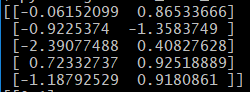This is 2 classes label data. The following ones are first 5 data.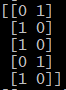This is 3 classes label data. The following ones are first 5 data.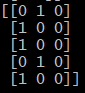This label data is created by setting 1 to only element atindex in the target vector. This method is called "1-of-K coding scheme".
The following left side figure is 2 classification sample data plot. The following right side one is 3 classification sample data plot.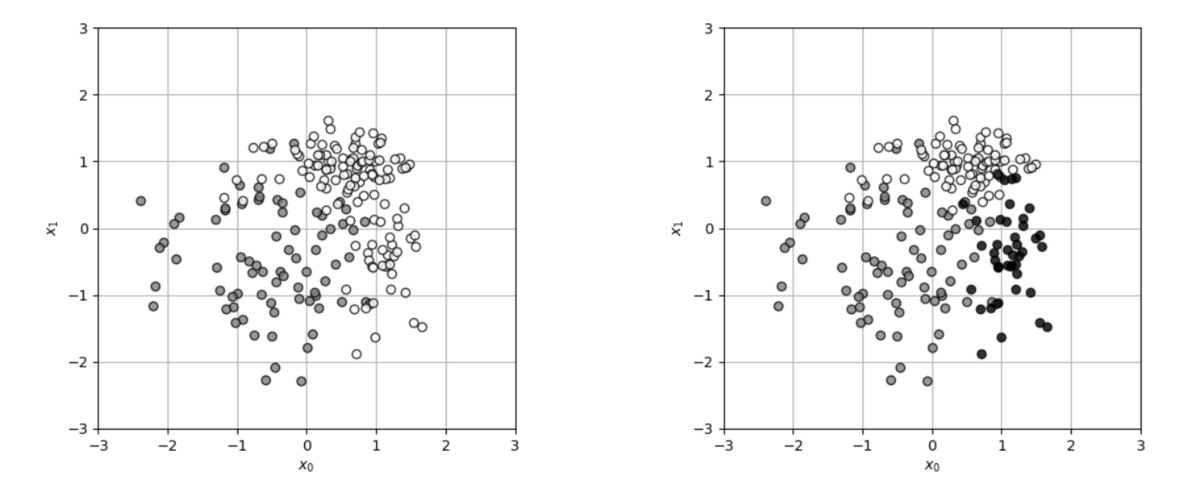## 2 classes classification

### Logistic regression model on 2 dimension

This is a 2 dimensional logistic regression model. An output of this modelis which approximates a probability.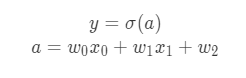The following 2 figures are the output of this model in the case of].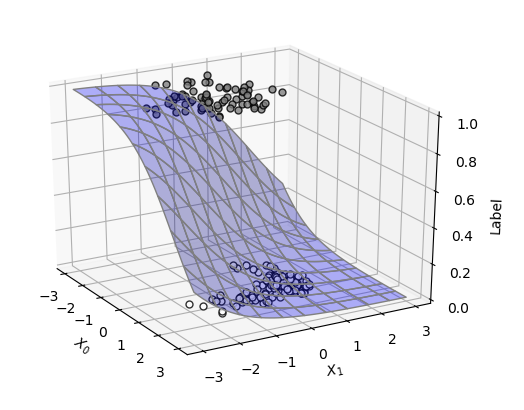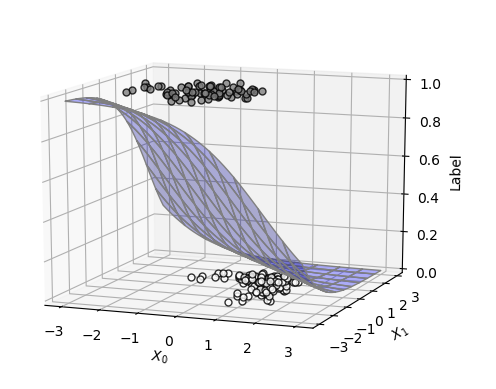### Mean cross entropy error

This following function can be used for mean cross entropy error same as the case of 1 dimension.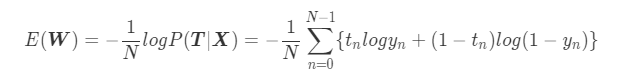And then, the partial derivative of this function can be calculated as follow.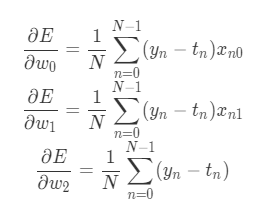### Calculating parameter by Gradient method

The following 2 figures of fitting result with 2 dimensional logistic regression model.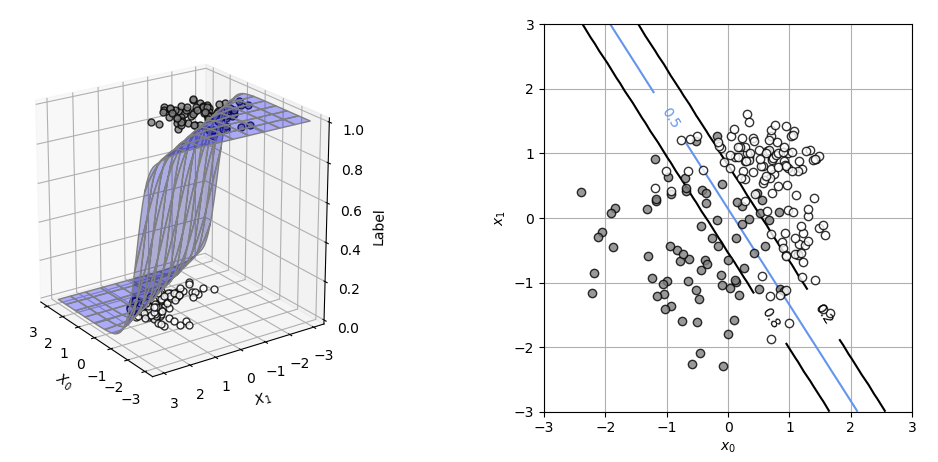The parameterswas calculated by "Conjugate gradient method". According to the above right side figure, an accurate decision boundary was created with those parameters.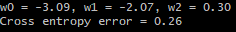## 3 classes classification

### Logistic regression model for 3 classes classification

This regression model is defined with Softmax function.
www.eureka-moments-blog.com
Total inputis defined as follow.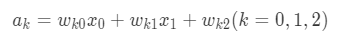And then, by assuming the 3rd input, this formula is deformed as follow.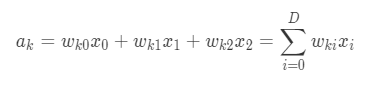This total input is used as input of the softmax function. An exponential functionand the total of exponential function at each classare defined.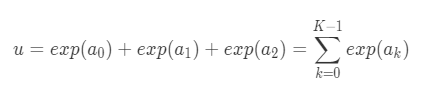is the number of class. In this case,. An output of softmax function is expressed with the aboveas follow.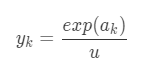An output of this model is]. And then,. The parameter of model is expressed as the following matrix.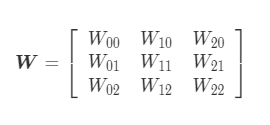Each output,,is expressed as a probability which the inputbelongs to each class as follow.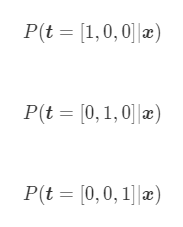### Mean cross entropy error

"Likelihood" is a probability which all of class datais generated for all of input data. This is expressed as follow.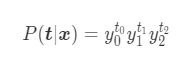The probability which all of label data was generated is calculated by the following formula.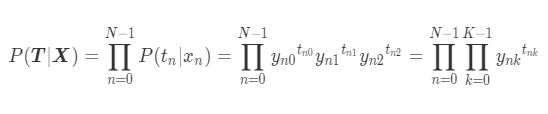According the above formula, an mean cross entropy error is defined as follow.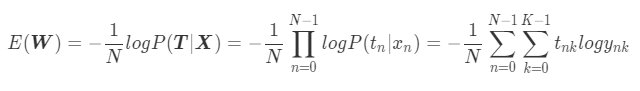### Calculating parameter by Gradient method

To calculatewhich minimizesby Gradient method, a partial derivative of eachis used.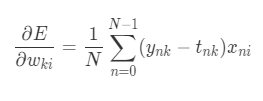The calculated parametersis this.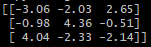The following figure is a fitting result by the logistic regression model with the above parameters.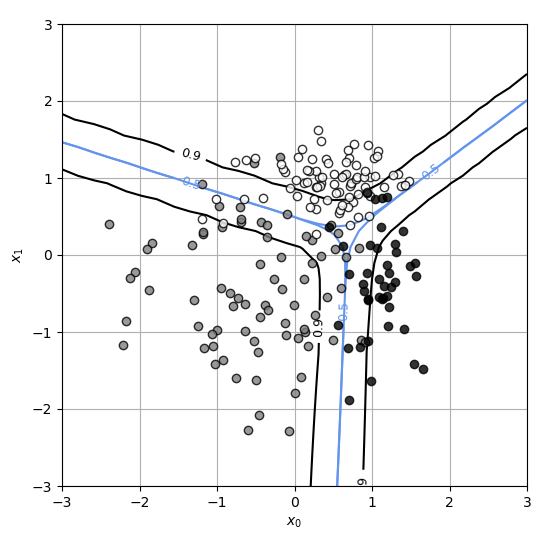And then, the cross entropy error is 0.26.

## Question

What is an advantage of using Cross entropy error function as a loss function?